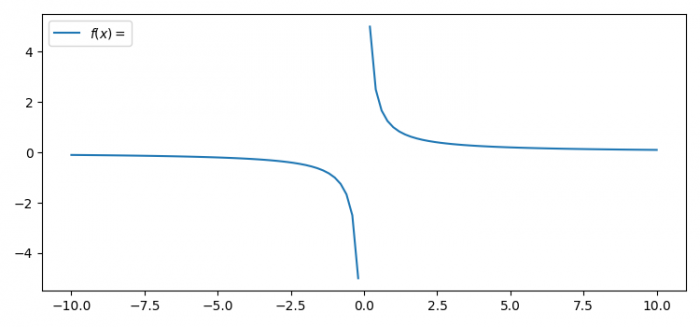# How to plot y=1/x as a single graph in Python?

To plot y=1/x as a single graph in Python, we can take the following steps −

• Set the figure size and adjust the padding between and around the subplots.
• Create data points using numpy.
• Plot x and 1/x data points using plot() method.
• Place a legend on the figure.
• To display the figure, use show() method.

## Example

import numpy as np
import matplotlib.pyplot as plt

plt.rcParams["figure.figsize"] = [7.50, 3.50]
plt.rcParams["figure.autolayout"] = True

x = np.linspace(-10, 10, 101)
plt.plot(x, 1/x, label='$f(x)=\frac{1}{x}$')
plt.legend(loc='upper left')

plt.show()

## Output